Case Based Questions Test: Probability

# Case Based Questions Test: Probability - Class 10

Test Description

## 10 Questions MCQ Test Mathematics (Maths) Class 10 - Case Based Questions Test: Probability

Case Based Questions Test: Probability for Class 10 2023 is part of Mathematics (Maths) Class 10 preparation. The Case Based Questions Test: Probability questions and answers have been prepared according to the Class 10 exam syllabus.The Case Based Questions Test: Probability MCQs are made for Class 10 2023 Exam. Find important definitions, questions, notes, meanings, examples, exercises, MCQs and online tests for Case Based Questions Test: Probability below.
Solutions of Case Based Questions Test: Probability questions in English are available as part of our Mathematics (Maths) Class 10 for Class 10 & Case Based Questions Test: Probability solutions in Hindi for Mathematics (Maths) Class 10 course. Download more important topics, notes, lectures and mock test series for Class 10 Exam by signing up for free. Attempt Case Based Questions Test: Probability | 10 questions in 20 minutes | Mock test for Class 10 preparation | Free important questions MCQ to study Mathematics (Maths) Class 10 for Class 10 Exam | Download free PDF with solutions
 1 Crore+ students have signed up on EduRev. Have you?
Case Based Questions Test: Probability - Question 1

### Read the following text and answer the following questions on the basis of the same:On a weekend Rani was playing cards with her family. The deck has 52 cards.If her brother drew one card.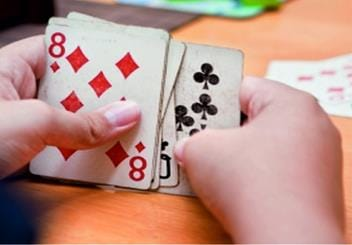Find the probability of getting a king of red colour.

Detailed Solution for Case Based Questions Test: Probability - Question 1
No. of cards of a king of red colour = 2

Total no. of cards = 52

Probability of getting a king of red colour

= No. of king of red colour/Total number of cards

= 2/52 = 1/26

Case Based Questions Test: Probability - Question 2

### Read the following text and answer the following questions on the basis of the same:On a weekend Rani was playing cards with her family. The deck has 52 cards.If her brother drew one card.Find the probability of getting a face card.

Detailed Solution for Case Based Questions Test: Probability - Question 2
Total number of cards = 52

Face cards are King, Queen and Jack

Each type has 4 cards

Total number of face cards = 3 x 4 = 12

P (getting a face card) = Number of face cards/Total number of card

= 12/52

= 3/13

Case Based Questions Test: Probability - Question 3

### Read the following text and answer the following questions on the basis of the same:On a weekend Rani was playing cards with her family. The deck has 52 cards.If her brother drew one card.Find the probability of getting a jack of hearts.

Detailed Solution for Case Based Questions Test: Probability - Question 3
Total number of cards = 52

We have 4 Jack cards

Total number of Jack of hearts = 1

P (getting a face card) = Number of jack of heart cards/Total number of cards

= 1/52

Case Based Questions Test: Probability - Question 4

Read the following text and answer the following questions on the basis of the same:

On a weekend Rani was playing cards with her family. The deck has 52 cards.If her brother drew one card.Find the probability of getting a red face card.

Detailed Solution for Case Based Questions Test: Probability - Question 4

No. of red face card = 6

Total no of cards = 52

Probability of getting a face card

= No. of red face cards/Total no. of cards

= 6/52 = 3/26

Case Based Questions Test: Probability - Question 5

Read the following text and answer the following questions on the basis of the same:

On a weekend Rani was playing cards with her family. The deck has 52 cards.If her brother drew one card.

Find the probability of getting a spade.

Detailed Solution for Case Based Questions Test: Probability - Question 5

No. of spade card = 13

Total no of cards = 52

Probability of getting a spade card

= No. of spade cards/Total no. of cards

= 13/52 = 1/4

Case Based Questions Test: Probability - Question 6

Read the following text and answer the following questions on the basis of the same:

Rahul and Ravi planned to play Business (board game) in which they were supposed to use two dice.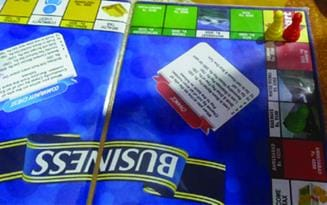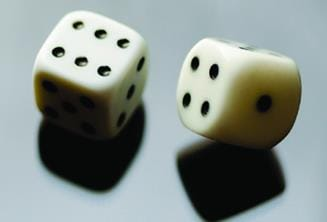Ravi got the first chance to roll the dice. What is the probability that he got the sum of the two numbers appearing on the top face of the dice is 8?

Detailed Solution for Case Based Questions Test: Probability - Question 6
The outcomes when two dice are thrown together are

= (1, 1), (1, 2), (1, 3), (1, 4), (1, 5), (1, 6)

(2, 1), (2, 2), (2, 3), (2, 4), (2, 5), (2, 6)

(3, 1), (3, 2), (3, 3), (3, 4), (3, 5), (3, 6)

(4, 1), (4, 2), (4, 3), (4, 4), (4, 5), (4, 6)

(5, 1), (5, 2), (5, 3), (5, 4), (5, 5), (5, 6)

(6, 1), (6, 2), (6, 3), (6, 4), (6, 5), (6, 6)

Total outcomes = 36

No. of outcomes when the sum is 8 = 5

Probability = 5/36

Case Based Questions Test: Probability - Question 7

Read the following text and answer the following questions on the basis of the same:

Rahul and Ravi planned to play Business (board game) in which they were supposed to use two dice.Rahul got the next chance. What is the probability that he got the sum of the two numbers appearing on the top face of the dice is 13?

Detailed Solution for Case Based Questions Test: Probability - Question 7
No. of outcomes when the sum is 13 = 0

Total outcomes = 36

Probability = 0/35 = 0

Case Based Questions Test: Probability - Question 8

Read the following text and answer the following questions on the basis of the same:

Rahul and Ravi planned to play Business (board game) in which they were supposed to use two dice.Now it was Ravi’s turn. He rolled the dice. What is the probability that he got the sum of the two numbers appearing on the top face of the dice is less than or equal to 12 ?

Detailed Solution for Case Based Questions Test: Probability - Question 8
No. of outcomes when the sum is less than or equal to 12 = 36

Total outcomes = 36

probability = 36/36 = 1

Case Based Questions Test: Probability - Question 9

Read the following text and answer the following questions on the basis of the same:

Rahul and Ravi planned to play Business (board game) in which they were supposed to use two dice.Rahul got next chance. What is the probability that he got the sum of the two numbers appearing on the top face of the dice is equal to 7 ?

Detailed Solution for Case Based Questions Test: Probability - Question 9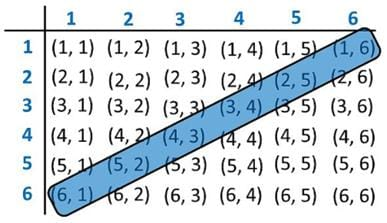Number of outcomes where sum is 7 = 6

Probability that sum of two numbers is 7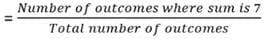= 6/36

= 1/6

Case Based Questions Test: Probability - Question 10

Read the following text and answer the following questions on the basis of the same:

Rahul and Ravi planned to play Business (board game) in which they were supposed to use two dice.Now it was Ravi’s turn. He rolled the dice. What is the probability that he got the sum of the two numbers appearing on the top face of the dice is greater than 8 ?

Detailed Solution for Case Based Questions Test: Probability - Question 10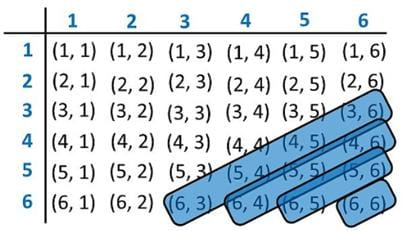Number of outcomes where sum is greater than 8

= 4 + 3 + 2 + 1

= 10

Probability that sum of two numbers is greater than 8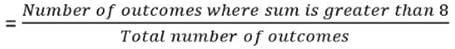= 10/36

= 5/18

## Mathematics (Maths) Class 10

115 videos|479 docs|129 tests
Information about Case Based Questions Test: Probability Page
In this test you can find the Exam questions for Case Based Questions Test: Probability solved & explained in the simplest way possible. Besides giving Questions and answers for Case Based Questions Test: Probability, EduRev gives you an ample number of Online tests for practice

## Mathematics (Maths) Class 10

115 videos|479 docs|129 tests